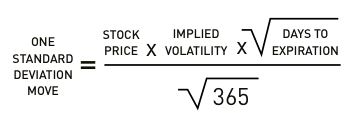# How can I calculate 1 standard deviation move over the life of an options contract?

Standard Deviation (SD) is a unit of measurement that expresses how much members of a group deviate from its mean value. In other words, it quantifies how outcomes compare to the average outcome.

In the case of options contracts:For example, ITC shares are trading at Rs 200 with an Implied Volatility (IV) of 20% and 30 days to expiry.

Hence, 1 SD move
= ( 200 * 20% * square root of 30 ) / square root of 365
= 11.47

Based on its current IV, a stock generally ends up within:
• 1 standard deviation of its original price 68% of the time,
• 2 standard deviations of its original price 95% of the time, and
• 3 standard deviations of its original price 99% of the time.

Still need help?